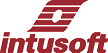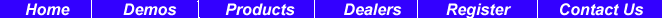Newsletter Issue #60, June 2000

# Scope 5 New Features

The third beta release of Scope5 is now available for download from the web (http://www.intusoft.com/products/scope/scope8pic.htm). The IsSpice Interactive Control Language, ICL, that is shared with Scope5 has been expanded to perform the following Scope4 functions:

1. Fourier transforms and
inverse Fourier transforms

2. Wavelet and inverse
wavelet transforms

3. Polynomial regression

4. Piece-wise linear
constructs

5. Complex functions
6. Building independent
waveforms

In this release, you can access HTML help for the calculator functions by using the help cursor to select the menu items. You can also use <shift + F1> to get thehelp cursor. We bring up an HTML page with boilerplate navigation so you can drill down into the ICL. Each script has a description, example and script listing of the item. To make your own function, you just add the .scp and .html files to the scripts folder. In the next update we will add bitmap files that correspond to the .scp file and make provisions for you to customize your tool bar in order to have single click access to any script. The following is a reproduction of the help information for the Black Body script along with its result.

 In This Issue 1 Scope 5 New Features 7 New IsSpice Option Improves Convergence 11 New IsSpice Reporting 11 Preview New SpiceMod for Windows 14 Using VB Scripts with IsSpice, Revisited

# Black Body

Description

Generate and plot black-body radiation curves in the visible spectrum for 3200, 6000 and 9000 deg K.

This script illustrates many ICL features. First it loads rule.plt; the defining plot for dimensional (units) analysis. This is a user extensible file that defines the relationship between basic units; for example watt = volt*amp. It is a special plot in which units are not evaluated. The units are then copied into the constants plot so that they can be used to define units for the various constants we will use for this problem. Constants are then defined along with their units. We set the angle computation to radians and reset it at the end to degrees. The function eb(t,u), is the black body radiation function taken from a text book. The frequency, u, will be passed into the function as a vector. That forces the left hand side of the equation to also be a vector of the same length. What that means to you, the user, is that the expression evaluation will automatically loop through each wavelength using an efficient C language coded loop that runs thousands of times faster than an equivalent interpretive language like Visual Basic.

The vector function is built into the ICL language and creates a vector from 0 to the magnitude of its argument, with the number of elements equal to the argument. “wavelength” is then the x-axis scale for the blackbody plot, running from 400 nano meters to (400+380) nano meters; the visible specrum of light. Newplot and setplot make the plot; copying wavelength over as the default vector. The keyword “default” can then refer to the wavelength. “c/default” is evaluated to be a vector and its vector property causes the function eb() to be evaluated as a vector for each of the wavelengths. Its then a simple matter to plot each curve, normalized to its own mean. Dimensional analysis proceeds as you go so that dimensional errors will wind up with strange or unknown units.

#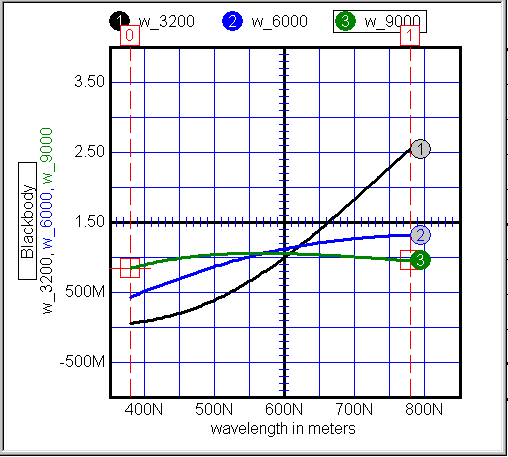Figure 1. Black-body radiation curves show ICL features.

### Script:

nv = nextvector(null)
while nv != null
constants.nv = nv
nv = nextvector(nv)
end
setplot constants
h = 6.62607e-034 * joule * time
k = 1.38e-23 * joule / kelvin
c = 300meg * length / time
e = exp(1)
function eb(t,u) ((2 * pi * h * u^3)/(c^2
(e^((h * u) / (k *t)) - 1)))
print h k c pi e
wavelength = length*(vector(100)/99*400n+380n)
newplot blackbody wavelength
setplot blackbody
w_3200 = eb(3200*kelvin,c/default)
w_3200 = w_3200/mean(w_3200)
plot w_3200
w_6000 = eb(6000*kelvin,c/default)
w_6000 = w_6000/mean(w_6000)
plot w_6000
w_9000 = eb(9000*kelvin,c/default)
w_9000 = w_9000/mean(w_9000)
plot w_9000
set units = deg
Example:
This script produces a standalone result.

Hot Key: None

# Calculating Units

As shown in the Black Body example, Scope5 also performs dimensional analysis as the expression evaluation proceeds. The capability is greatly enhanced from Scope4. Each quantity can have units specified in string format. Any string is acceptable; however, only strings defined in the rule.plt file are simplified. Units are defined as a polynomial ratio of products of string names. When illegal operations are requested such as sum = 5*apples + 3*oranges, the math is performed and the resulting units are called unknown. Any further expressions involving unknown units will also be unknown. Expressions that evaluate with unknown units may be in error.

Polynomial units in Scope5:
 amps joules coulombs volts deg db hertz secs “volt volts/hertz” ohms db(volts) watts db (amps)

The unit polynomials are compressed in the order they are listed in rule.srt. The file, rule.unt is a text file that describes additional units that can appear in the “Trace Properties” dialog (File menu). You can attach units using the dialog to reduce the chance of making a clerical mistake with the set units command.

 You can add additional units by placing them in rule.unt as follows: The “rule.unt” file must be a comma and line delimited text file, where each line defines a separate unit. Each line must contain three fields (1) the unit name, which will appear in the selection lists, (2) the unit string used by Units, and (3) any operator needed by Units (this field may be empty, but a comma is required). Example: “db(volts/sec^2), volts/(sec*sec), db”.A convenient way to attach units is to copy rule.plt into constants as shown in the Black Body script. Then you can attach units to a quantity by multiplying by the appropriate descriptor; for example v = 35 * volt. Will print out as 35.0 volts. In the future we will link the schematic units to the IsSpice plots and define units within libraries so that non-electrical systems will appear seamless:

# Pan and Scroll Bars

 Long traces present special challenges for a waveform viewer. The data from the snubber drawings in Figure 2 is an example of a plot of a periodic waveform that just shows up as a blob when viewing the entire waveform. You need to zoom into the waveform to see the detail; however, you still need to have some idea of where you are in the overall context. The Scope4 and Scope5 scaling dialog lets you see the context. In Scope5 we have added a pan and scale scrollbars that are much easier to control than the bounding box. The size of the pan scrollbar matches the section of the waveform to view and the location of the scale scrollbar marks the end of the expanded trace. It works pretty much the way the knobs do on an oscilloscope, except the control is linear instead of circular. Results shown in Figure 2 illustrate these features.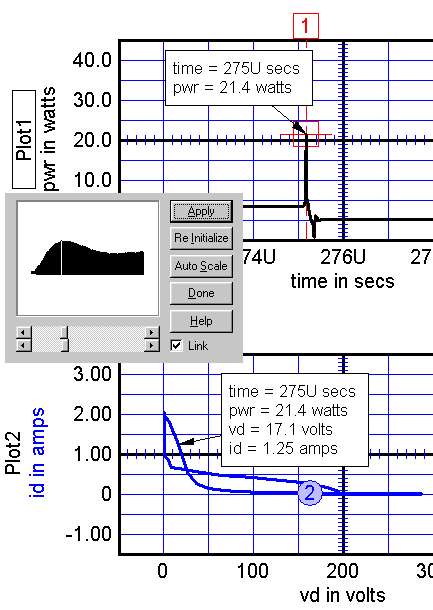Figure 2. Detailed view of periodic snubber waveform.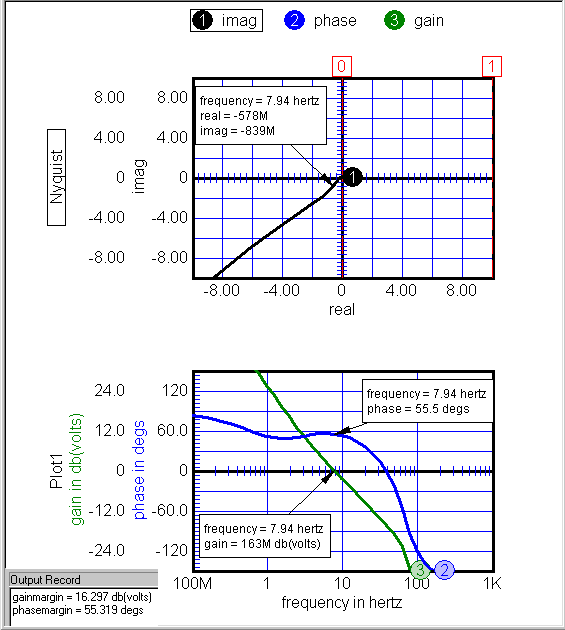Figure 3. Viewing the labeling of a Nyquist plot from a servo drawing.
 Parametric Cursors and Labels When a graph has more than 1 plot, for example power vs. time and current vs voltage, then the scales can be linked. Linkage has 2 benefits: 1. The slave trace is visible only for points that are visible in the master trace. This isolates the slave trace view to eliminate clutter. 2. Cursors in the master trace produce labels in the slave trace that point to the appropriate x-y value thereby forming a parametric cursor that advances along a double valued path. Figure 3 illustrates the technique for viewing the labeling of a Nyquist plot from a servo drawing. Figure 4 shows a behavioral model of a simple servo system.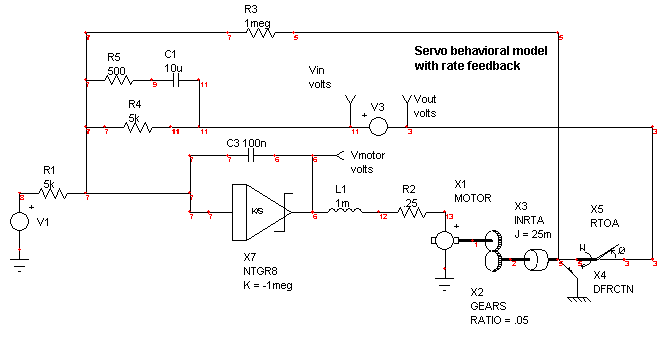Figure 4. A behavioral model of a simple servo system.

# Improves Convergence

 Numerical convergence in Spice simulators is closely coupled to the concept of the error in numerical integration. Reducing the time step reduces numerical integration errors. The error boundary for the numerical integration is estimated and is the basis for setting the transient step time. For many circuits, simulations contain sharp voltage steps that are not constrained by numerical integration. When this occurs, integration accuracy is unaffected so that the simulator is allowed a large time step. This results in a loss in accuracy because the excessively large time steps can jump over needed details. In the past, it was necessary to restrict this behavior by setting Tmax in the .TRAN statement. The January 2000 newsletter (http://www.intusoft.com/nl59.htm) addressed this problem using the available simulation techniques. The problems arising out of the circuit used for the January 2000 newsletter caused us, here at Intusoft, to explore IsSpice code changes that can solve the problem in a more efficient manner. It turns out there was a code fragment left over from an earlier attempt by us to solve the problem, but was not implemented because we tried to reduce the time step until a specified voltage accuracy was achieved; an impossible task for a switching waveform. Modifying the constraint, on the other hand, to test for a volt-second error produces the desired result. The latter method will always find a time step that is small enough while the earlier method will have a constant error as the time step is reduced. We introduced a new option, VSECTOL, which reduces the time step if the product of the absolute value of the error in predicted voltage (prediction – solution) and the time step exceeds the VSECTOL specification. As in most IsSpice options, its default is zero, which turns the option off. In testing the new VSECTOL option, we ran some cases setting RELTO=0.5 and at the same time set VSECTOL to a reasonable value for the circuit. Figure 5 shows this new time step control option. Figures 6 and 7 show the results for a simplified power supply model versus the standard OPTIONS to control the time step. This introduces a completely new time step control for the simulation, based on node voltage accuracy. Using snubber.dwg, shown in Figure 5, as a test circuit we ran the usual analysis. All we needed to do was set VSECTOL=50n, RELTOL=.5 and BYPASS = OFF; no TMAX was needed in the .tran statement. On the other hand, many parameters were modified to get spice to run this circuit to completion, including setting TMAX to 20n. The VSECTOL controlled simulation ran faster and produced more detail in the switching transitions. The conventional simulation spent a lot of extra time when the circuit wasn’t switching and took fewer points in the switching transition. The Bypass option controls whether or not the device operating point is calculated for each time step. When Bypass is ON, these load operations are skipped if errors are low enough. Since RELTOL is used in the calculations, we can’t use the BYPASS logic.Figure 5. Snubber.dwg uses VSECTOL for time step control.

#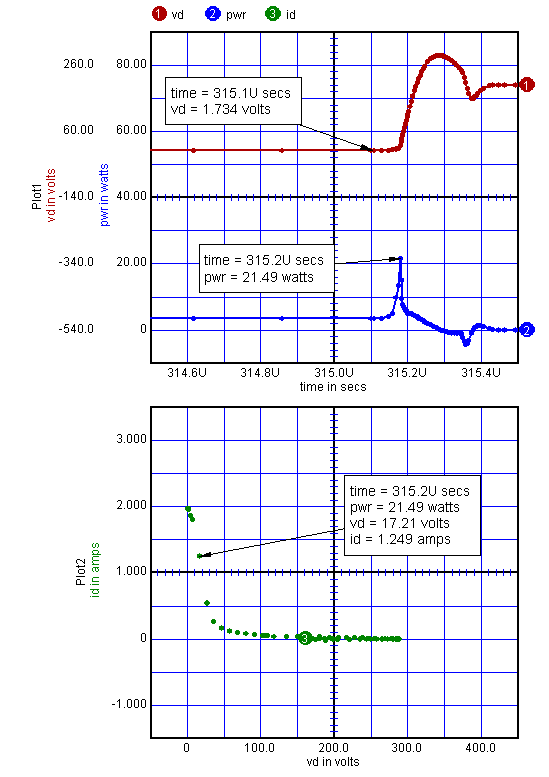Result using VSECTOL to control the time step produces higher accuracy during the turn-off transition and uses less computational resources when there is no switching activity. Transient timepoints = 26015 Accepted timepoints = 14217 Rejected timepoints = 11798 Total Analysis Time = 35.15

 Figure 6. A simplified power supply model for switching transistor stress test and snubber design.

#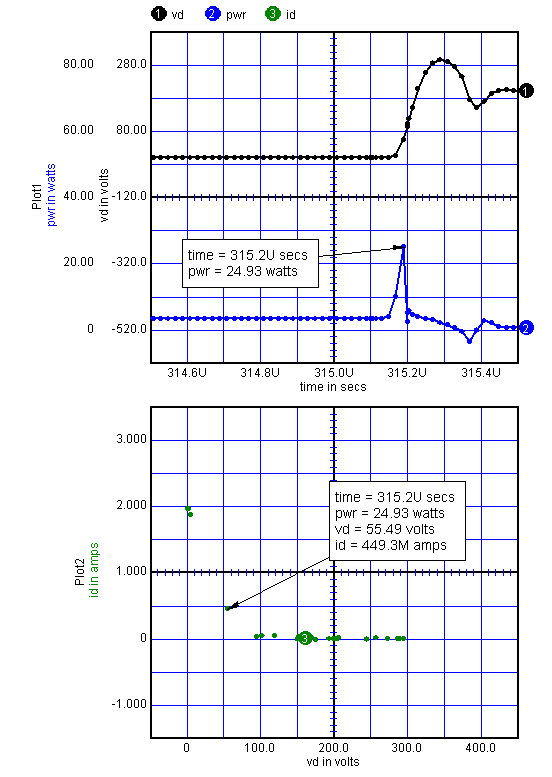Result using standard OPTIONS to control the time step produces lower accuracy during the turn-off transition and uses excessive computational resources when there is no switching activity. Transient timepoints = 51381 Accepted timepoints = 51339 Rejected timepoints = 42 Total Analysis Time = 48.033

 Figure 7. This power supply model uses standard OPTIONS versus VSECTOL for time step control.

# New IsSpice Reporting

 1. Element usage is now reported in the IsSpice “.out” file. The following is an example from snubber.dwg. ********* Parts Statistics******* CAPACITOR 5 RESISTOR 15 INDUCTOR 3 DIODE 5 BJT 1 MOSFET 1 V-SOURCE 7 B-SOURCE 5 VCVS 1 CCCS 2 *************************************** 2. Nodes with less than 2 connections are noted with a warning in the output. Failure to connect ground, node 0, to anything will produce this warning.

# Preview New SpiceMod for Windows

 Intusoft is coming out with a new Windows-based Spicemod program. Spicemod allows you to produce accurate spice models for use with any Berkeley SPICE compatible program. The models are stored in ASCII text format ensuring easy file transfer and editing. The generated models accurately simulate DC characterization, transient switching effects, capacitive effects, temperature variations, and power dissipation. Spicemod can produce very accurate models with a minimum amount of data. As you enter data the program dynamically updates the other data sheet values to give you an even more accurate model. This feature ensures that you get a model with reasonable DC, AC, and transient behavior even if you enter the minimum values needed. Of course the more data you enter the more accurate your model becomes. SpiceMod is designed for the working engineer and allows the user to quickly and easily develop maximum, minimum, typical, and worst case models. After the user creates and saves his model he is still able to go back to the device data enter screen and see his previously entered data. This enables the user to quickly make variations of his device by just modifying the desired parameters. Spicemod5 for Windows gives you a new graphical interface (see Figure 8), new model types and more accurate models. If you have Intusoft’s ICAP/4Windows software you are able to instantly test out the models generated.

 SpiceMod Capabilities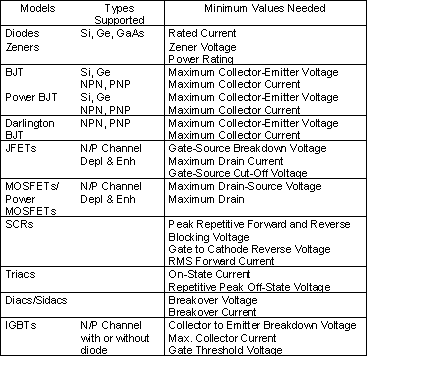SpiceMod is designed for the working engineer and allows the user to quickly and easily develop maximum, minimum, typical, and worst case models. After the user creates and saves his model he is still able to go back to the device data enter screen and see his previously entered data. This enables the user to quickly make variations of his device by just modifying the desired parameters. Spicemod5 for Windows gives you a new graphical interface (see Figure 8), new model types and more accurate models. If you have Intusoft’s ICAP/4Windows software you are able to instantly test out the models generated.
 How SpiceMod Works SpiceMod understands both data sheet parameters and SPICE model parameters. It also understands how to convert from one to the other. As data sheet values are entered, they are immediately analyzed and the proper conversions to the SPICE model or SPICE subcircuit parameters are displayed. The more data you enter, the more accurate the model will be. However, if only limited data is available, SpiceMod will make estimates for the remaining data based on the data you have entered. Custom Subcircuits Some common semiconductors such as Power Mosfets and Power BJTs cannot be modeled with the basic SPICE .MODEL statement. A subcircuit approach using several elements must be used. Although some SPICE model vendors try, use of the .MODEL statement alone will not allow critical dynamic parameters and parasitics to be properly modeled. That’s why Intusoft has placed several custom designed subcircuit representations for these devices in SpiceMod.Figure 8. SpiceMod5's new Windows graphical user interface makes entering parameters easier.

# Using VB Scripts with IsSpice, Revisited

 Intusoft was the first SPICE program to offer OLE (Object Linking and Embedding) and Visual Basic interfaces. Basically OLE is a technology that allows one program, an OLE Controller, to take advantage of services provided by another program, an OLE Server. The computing features and functions exposed by the OLE Server are called OLE objects. An OLE interface essentially allows two programs to share functionality without having to know about one another. IsSpice4 supports two types of OLE enhancements. The first is that IsSpice4 is an OLE Server. This means that any OLE Controller can drive the simulator. For example, a math program, like MATLAB, could use IsSpice4 as a simulation engine and ask it to run an analysis and pass back the results so that they can be used in a subsequent MATLAB calculation. Secondly, IsSpice exposes an Interactive Command Language (ICL) as an accessible set of OLE objects. ICL is a scripting language that contains a wide variety of simulation related functions such as analysis commands and functions that change part values. A simple script, or macro, to run 2 simulations is:

 view tran v(3) (Display V(3) as the simulation runs) tran 1n 200n (Run a transient analysis for 200ns) print v(3) (Send the data for V(3) to the OLE Controller) alter @r1[resistance]=@r1[resistance]*1.10 (Increment R1 10%) tran 2n 300n (Run another longer transient analysis for 300ns print) v(3) (Send the data for V(3) to the OLE Controller)

 The ICL is a publicly available protocol enhanced by Intusoft to interface external applications to the IsSpice4 simulator. See http://www.intusoft.com/script/pages/index.htm for more information on this powerful scripting language. It exposes all of the interactive functionality of IsSpice4. With OLE, the ICL interface is distilled into a form that makes it easy to access all of the capabilities of SPICE. Additional OLE interfaces to control the IntuScope post processor and to implement schematic cross-probing features can also be obtained from the IsSpice4 OLE interface. To demonstrate IsSpice4’s OLE interface, we will use a Visual Basic (VB) Script (Figure 9) to start IsSpice4 and remotely run Transient and Fourier analyses. In this case, the script is run from the Microsoft Excel spreadsheet program. The simulation uses values taken from the spreadsheet. The IsSpice4 results are returned to the spreadsheet. The SPICE.DoScript command sends ICL functions to IsSpice4. Several ICL statements can be concatenated into one DoScript or sent individually. In the above VB script, 3 transient simulations are run. For each analysis the value of the resistor (RF) is set based on the Excel spreadsheet (column A, rows 2:4) values using the strAlter= line. The total harmonic distortion results of a Fourier analysis are returned in SPICE.ScriptOutput and displayed in column B, rows 2:4. Many other more complex applications are possible. IsSpice4 is the ONLY SPICE simulator to offer OLE and Visual Basic interfaces. It is just another way in which Intusoft distinguishes itself from other EDA tool vendors. Figure 9 demonstrates how a Visual Basic script interfaces with an Excel spreadsheet. The SPICE.DoScipt command sends ICL functions to IsSpice4. Several ICL statements can be concatenated into one DoScript or sent individually. In the above VB script, 3 transient simulations are run. For each analysis, the value of the resistor (RF) is set based on the Ecel spreadsheet (column A, rows 2:4) values using the strAlter=line. The total harmonic distortion results results of a Fourier analysis are returned in SPICE.ScriptOutput and displayed in column B, rows 2:4.

 VBScript.txt vSub ICAPS( ) Dim ICAPS As Object Dim SPICE As Object Dim i As Integer Dim j As Integer Dim k As Integer Dim str As String Dim strOutput As String Dim pos1 As Integer Dim pos2 As Integer Dim strTHD As String 'creates the ICAPS Object Set ICAPS = CreateObject("ICAPS.Application.1") 'set the circuit name ICAPS.CircuitFullName = "c:\spice8\circuits\vbdrawing.cir" ' run a simulation ICAPS.ForceSimulate ' keep looking until we find that IsSpice has launched j = ICAPS.SpiceStartStatus While j < 1 j = ICAPS.SpiceStartStatus Wend If j = 2 Then str = "An error occurred starting IsSpice" GoTo Quit End If ' Get the IsSpice object On Error Resume Next Set SPICE = GetObject (, "IsSpice4.Application.1") If Err > 0 Then str = "IsSpice4 is busy or not running." GoTo Quit Else str = SPICE.Caption End If ' loop until we are ready to accept scripts or IsSpice has gone away k = SPICE.ScriptStatus j = ICAPS.SpiceStartStatus If j = 0 Then str = "IsSpice quit." GoTo Quit End If While k = 0 k = SPICE.ScriptStatus j = ICAPS.SpiceStartStatus If j = 0 Then str = "IsSpice quit." k = 2 End If Wend If j = 0 Then str = "IsSpice quit." GoTo Quit End If If j = 2 Then str = "An error occurred running IsSpice." GoTo Quit End If ' This is the heart of the VB macro ' We read data from the Data sheet run a TRAN and FOURIER then print the THD to the ' Results sheet For i = 2 To 4 Step 1 SPICE.DoScript "setplot tran" SPICE.DoScript "alter @rf[resistance]=" +Worksheets("Data").Range("a" + CStr(i)).Value SPICE.DoScript "run start" SPICE.DoScript "fourier 10k v(3)" strOutput = SPICE.ScriptOutput pos1 = InStr(strOutput, "THD:") pos2 = InStr(strOutput, "%") strTHD = Mid(strOutput, (pos1 + 4), pos2 - (pos1 + 4)) Worksheets("THD").Range("b" + CStr(i)).Value = strTHD Next i Quit ' Use this MsgBox to stop the script 'MsgBox str ICAPS.Quit Set ICAPS = Nothing 'This does not close the application Set SPICE = Nothing 'This does not close the application End Sub
 Figure 9. Visual Basic Script that drives IsSpice from Excel.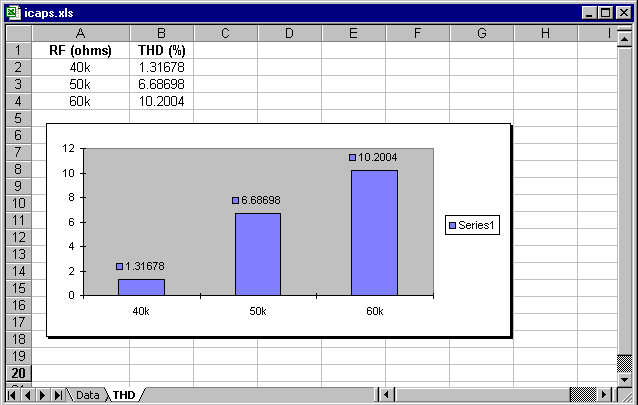Figure 10. A Visual Basic script, shown in Figure 9, is used to interface and Excel spreadsheet with IsSpice4. The spreadsheet shows the value of resistor RF and the resulting harmonic distortion of the amplifier circuit (inset). The VB script passes the RF values, runs the simulation, and returns the results. A graph of the THD is also shown. Go to http://www.intusoft.com/script/pages/VBscript.htm to test run this actual spreadsheet with IsSpice. The default path to vbdrawing.cir is c:\spice8\circuits.

BACK TO THE TOP